## Cognitive Study¶

### Elan Ding¶

Modified: June 30, 2018

I have combined all data into a single csv file called cognition.csv.

In :
import pandas as pd
import numpy as np
import matplotlib.pyplot as plt
import seaborn as sns
%matplotlib inline

In :
df = pd.read_csv('cognition.csv')


The first five rows of the data is displayed here. (Note I reversed the orientation so it fits better on the page.) In bold we have all the variables. The variable Cog Score is the mean cognition score amont four different sets of questions conducted in one day for each subject.

In :
df.head().transpose()

Out:
0 1 2 3 4
PIN 5191 7368 346 4116 5191
Cog Score 36 49.25 51.5 63.5 33.5
Visit 1 1 1 1 2
Age 72 77 52 72 72
Education 21 25 22 25 21
MothersEducation 16 16 16 27 16
Gender 2 2 2 2 2
Handedness 2 2 1 1 2
Race 1 1 1 1 1
Sequence C B D A D
Treatment Dx NaN Dx NaN RVDx4
Disease MM C MM C MM
Physical Score 7 6 6 1 11
Social Score 15 8 28 24 21
Economic Score 6 4 7 0 9
Functional Score 21 17 18 24 25
Additional Score 29 28 14 2 31
Total Score 78 63 73 51 97

## Exploratory Data Analysis¶

### Objective 1: Plotting cognitive scores and total QOL scores stratified by disease (MM vs Control).¶

In :
sns.lmplot(x='Cog Score', y='Total Score', hue='Disease', data=df)

Out:
<seaborn.axisgrid.FacetGrid at 0x7f302f9d6b70>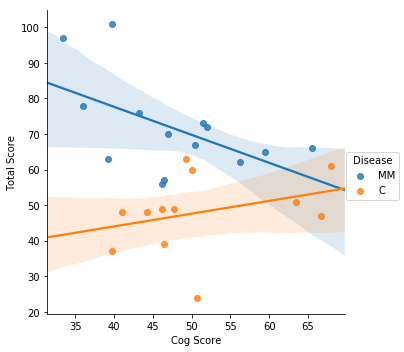It looks like that among MM patients, the cog score is inversely related to the QOL scores. For the control the relationship is opposite. This makes sense.

### Objective 2: Does sequence affect cognitive scores?¶

In :
sns.boxplot(x = 'Sequence', y='Cog Score', data=df)

Out:
<matplotlib.axes._subplots.AxesSubplot at 0x7f302f2f7278>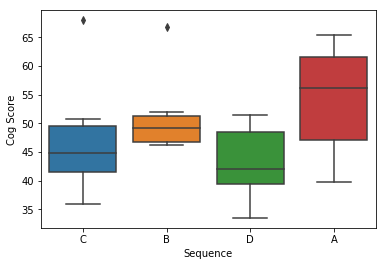Looks like that sequence A gives higher score. This is just a minor observation.

### Objective 3: Is gender a significant factor?¶

In :
sns.countplot(df['Gender'].astype(str), data=df)

Out:
<matplotlib.axes._subplots.AxesSubplot at 0x7f302f2f7630>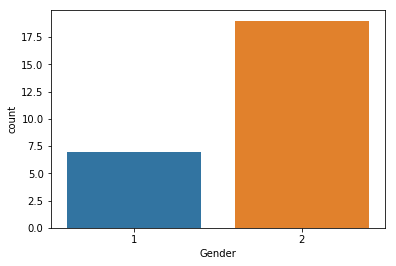In :
sns.boxplot(x = 'Gender', y='Cog Score', data=df)

Out:
<matplotlib.axes._subplots.AxesSubplot at 0x7f302f1849b0>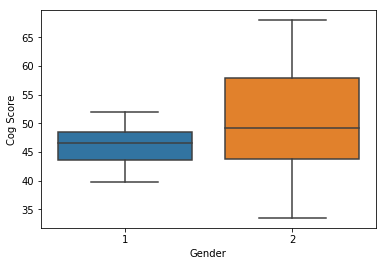In :
sns.boxplot(x = 'Gender', y='Cog Score', hue='Disease', data=df)

Out:
<matplotlib.axes._subplots.AxesSubplot at 0x7f302f281978>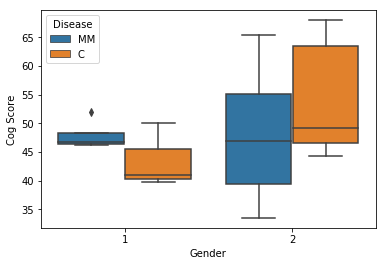In :
sns.boxplot(x = 'Gender', y='Total Score', data=df)

Out:
<matplotlib.axes._subplots.AxesSubplot at 0x7f302f092320>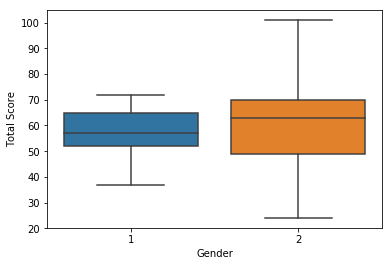It appears that gender is not a very significant factor.

### Objective 4: Is there a difference between MM patients and control?¶

In :
sns.countplot(df['Disease'].astype(str), data=df)

Out:
<matplotlib.axes._subplots.AxesSubplot at 0x7f302f076358>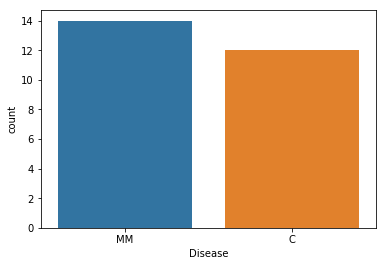In :
sns.boxplot(x = 'Disease', y='Cog Score', data=df)

Out:
<matplotlib.axes._subplots.AxesSubplot at 0x7f302efc3cf8>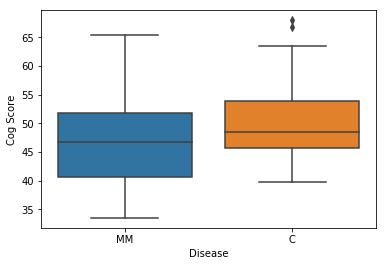In :
sns.boxplot(x = 'Disease', y='Cog Score', hue='Visit', data=df)

Out:
<matplotlib.axes._subplots.AxesSubplot at 0x7f302efba978>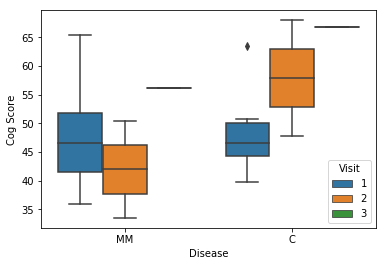Here we observe that among MM patients, the second visit gives a lower cognitive score, while among the controls, the second visit produced a higher score. (There is not enough data for the third visit yet.)

In :
sns.boxplot(x = 'Disease', y='Physical Score', data=df)

Out:
<matplotlib.axes._subplots.AxesSubplot at 0x7f302eeceac8>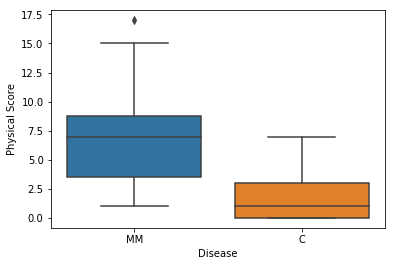In :
sns.boxplot(x = 'Disease', y='Physical Score', hue='Visit', data=df)

Out:
<matplotlib.axes._subplots.AxesSubplot at 0x7f302ee66ba8>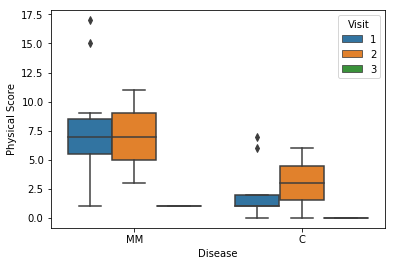In :
sns.boxplot(x = 'Disease', y='Social Score', data=df)

Out:
<matplotlib.axes._subplots.AxesSubplot at 0x7f302ed92da0>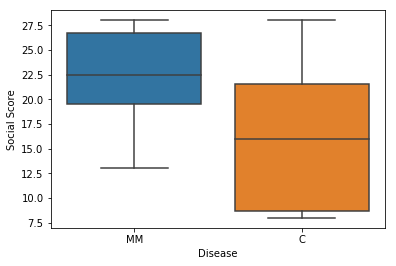In :
sns.boxplot(x = 'Disease', y='Social Score', hue='Visit', data=df)

Out:
<matplotlib.axes._subplots.AxesSubplot at 0x7f302ed9ae48>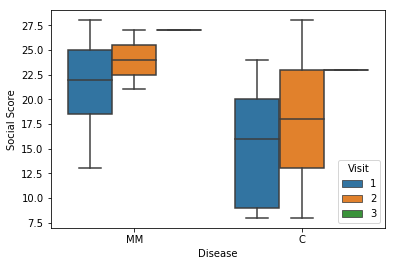In :
sns.boxplot(x = 'Disease', y='Economic Score', data=df)

Out:
<matplotlib.axes._subplots.AxesSubplot at 0x7f302ec67fd0>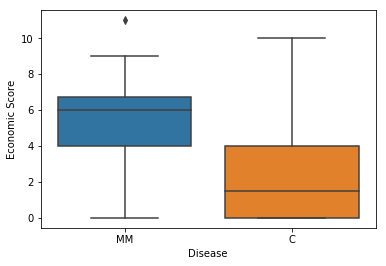In :
sns.boxplot(x = 'Disease', y='Economic Score', hue='Visit', data=df)

Out:
<matplotlib.axes._subplots.AxesSubplot at 0x7f302ebd33c8>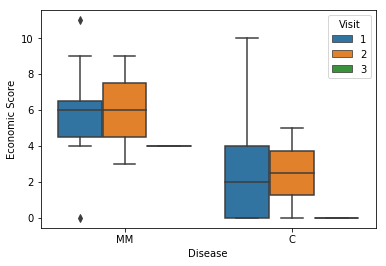In :
sns.boxplot(x = 'Disease', y='Functional Score', data=df)

Out:
<matplotlib.axes._subplots.AxesSubplot at 0x7f302eb13978>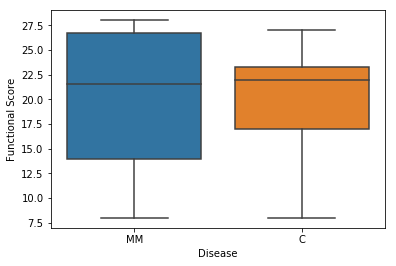In :
sns.boxplot(x = 'Disease', y='Functional Score', hue='Visit', data=df)

Out:
<matplotlib.axes._subplots.AxesSubplot at 0x7f302ea856d8>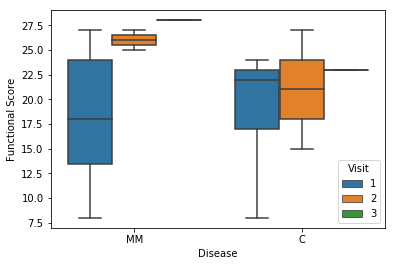In :
sns.boxplot(x = 'Disease', y='Additional Score', data=df)

Out:
<matplotlib.axes._subplots.AxesSubplot at 0x7f302e9c59b0>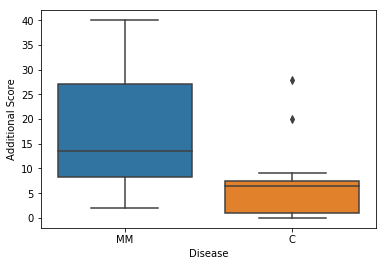In :
sns.boxplot(x = 'Disease', y='Additional Score', hue='Visit', data=df)

Out:
<matplotlib.axes._subplots.AxesSubplot at 0x7f302e9b0ac8>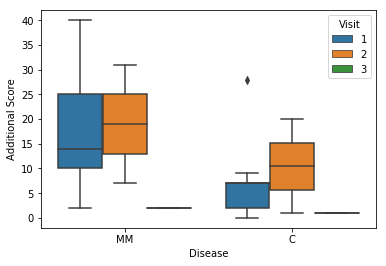In :
sns.boxplot(x = 'Disease', y='Total Score', data=df)

Out:
<matplotlib.axes._subplots.AxesSubplot at 0x7f302e8fd6a0>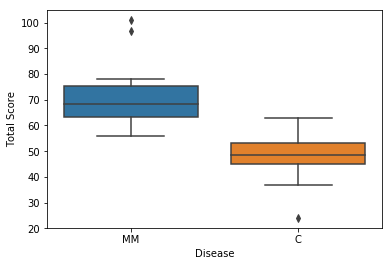In :
sns.boxplot(x = 'Disease', y='Total Score', hue='Visit', data=df)

Out:
<matplotlib.axes._subplots.AxesSubplot at 0x7f302e8732b0>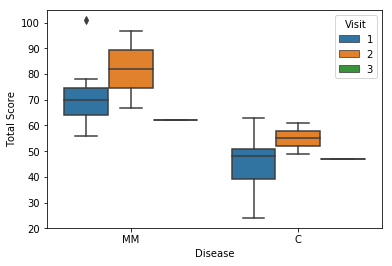Interestingly, we see an improvement between first and second visits in both MM and control groups.